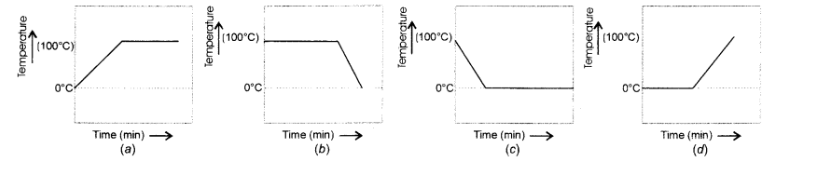# A student heats a beaker containing ice and water.`
Question:

A student heats a beaker containing ice and water. He measures the temperature of the content of the beaker as a function of time. Which of the following would correctly represent the result ? Justify your choice.Solution:

We know that the melting point of ice and the freezing point of water in pure states are both zero. This means that at this temperature, both are present. Upon heating, the temperature would actually not change but heat energy supplied would be absorbed by the ice as latent heat of fusion. This would result in the melting of ice. The temperature would remain zero degree till the whole of ice has melted. Further heating would increase the temperature of water till it starts boiling at $100^{\circ} \mathrm{C}$. The curve (d) gives the correct representaton.